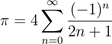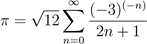# We Helped With This MATLAB Programming Homework: Have A Similar One?

SOLVEDCategory Programming MATLAB Undergraduate Solved Pay For Matlab Homework

## Assignment Description

MAE 8 - Fall 2017 Homework 6

Instructions: Follow the homework solution template. Put all answers in a MATLAB script named hw6.m. For this homework, you will need to submit multiple files. Create a zip archive named hw6.zip. The zip archive should include the following files: hw6.m, f.mat, and testpi.m. Submit hw6.zip through TritonED before 9 PM on 11/17/2017. Usedouble precision unless otherwise stated.

Problem 1: Italian mathematician Fibonacci is famous for introducing the 'Fibonacci series' to modern mathematics. Any term in the Fibonacci series is the sum of the previous two terms. For example, the first 5 terms of the series are

1,1,2,3,5

Use for loops (nested with if - break / continue statement when needed) in the following exercises.

(a)   Compute the first 50 terms of the series and put the answer in vector p1a.

(b)  In a single for loop, find the combined sum of the first 10 terms and the last 15 terms of the series in part (a). Put the answer in p1b.

(c)   Find the sum of the first 50 terms of the Fibonacci series excluding every fifth terms.Put the answer in p1c.

(d)  How many terms of the Fibonacci series are needed such that the sum of the termsis at least 90,000? Put the answer in p1d.

(e)   How many terms of the Fibonacci series are needed such that the product of theterms is at least 90,000? Put the answer in p1e.

Problem 2: Use function inv to solve for values of x’s in the following linear system of equations: A x = b. Note that the coefficient matrix A is tridiagonal. Put the answer in the 30-element vector p2. Hint: use for loop nested with if statement or function diag to construct the matrix.

 3x1 − x2 = 1 − x10 + 3x11 − x12 = 0 − x20 + 3x21 − x22 = 0 −x1 + 3x2 − x3 = 0 − x11 + 3x12 − x13 = 0 − x21 + 3x22 − x23 = 0 −x2 + 3x3 − x4 = 0 − x12 + 3x13 − x14 = 0 − x22 + 3x23 − x24 = 0 −x3 + 3x4 − x5 = 0 − x13 + 3x14 − x15 = 0 − x23 + 3x24 − x25 = 0 −x4 + 3x5 − x6 = 0 − x14 + 3x15 − x16 = 0 − x24 + 3x25 − x26 = 0 −x5 + 3x6 − x7 = 0 − x15 + 3x16 − x17 = 0 − x25 + 3x26 − x27 = 0 −x6 + 3x7 − x8 = 0 − x16 + 3x17 − x18 = 0 − x26 + 3x27 − x28 = 0 −x7 + 3x8 − x9 = 0 − x17 + 3x18 − x19 = 0 − x27 + 3x28 − x29 = 0 −x8 + 3x9 − x10 = 0 − x18 + 3x19 − x20 = 0 − x28 + 3x29 − x30 = 0 −x9 + 3x10 − x11 = 0 − x19 + 3x20 − x21 = 0 − x29 + 3x30 = −2

Problem 3: Download the file f.mat and load it into MATLAB. The file contains vector f. Without writing any loops or conditional statements, perform the following exercises.

(a)   Replicate each element in f 3 times and put the answer in p3a. For example, if f = [2 4 6], p3a should be [ 2 2 2 4 4 4 6 6 6]. Explore function repelem or the following statement: f(ceil((1:n*end)/n)) with n = 3.

(b)  Shift the elements in f 3 indices to the right and put the answer in p3b. For example, if f = [2 4 6 8], p3b should be [4 6 8 2]. Explore function circshift or the following statement: f(mod((1:length(f))-k-1,length(f))+1) with k = 3.

(c)   Find any values in f that are greater than 1 and less than 2, and replace them with 0. Put the answer in p3c. Vector p3c should have the same dimension as f.

(d)  Extract all values in f that are greater than 1 and less than 2 and put them in p3d in the same order as they appear in f.

Problem 4: Leibniz found that π can be approximated by the following series:.

Madhava (with Leibniz) later suggested an alternative series:.

In this exercise, you are asked to write a function testpi.m to compare how fast the two series can approximate the value of π for a given tolerance. The function should have the following declaration: function [api, nterm] = testpi(tol, method) where tol is the input tolerance defined as the absolute difference between the approximated π and the default value of π in MATLAB divided by the default value. Method is a string input being either 'Leibniz' or 'Madhava'. The function outputs are the approximated value of π api and the number of terms nterm in the series needed to compute the approximate value.

In the function, you may want to consider the relationship between abs(api-pi)/pi and tol as a condition to truncate n in the two series above. Give the function a description. In the following exercises, set the tolerance to 10−7.

(a) Set p4a=evalc('help testpi').

(b,c) For the Leibniz series, what is the approximated value of π and how many terms of the series are needed to compute that value? Put the answers in p4b and p4c, respectively.

(d,e) For the Madhava series, what is the approximated value of π and how many terms of the series are needed to compute that value? Put the answers in p4d and p4e, respectively. (f) Which method converges faster? Give answer in p4f = '... series converges faster'.

Is it free to get my assignment evaluated?

Yes. No hidden fees. You pay for the solution only, and all the explanations about how to run it are included in the price. It takes up to 24 hours to get a quote from an expert. In some cases, we can help you faster if an expert is available, but you should always order in advance to avoid the risks. You can place a new order here.

How much does it cost?

The cost depends on many factors: how far away the deadline is, how hard/big the task is, if it is code only or a report, etc. We try to give rough estimates here, but it is just for orientation (in USD):

 Regular homework \$20 - \$150 Advanced homework \$100 - \$300 Group project or a report \$200 - \$500 Mid-term or final project \$200 - \$800 Live exam help \$100 - \$300 Full thesis \$1000 - \$3000

How do I pay?

Credit card or PayPal. You don't need to create/have a Payal account in order to pay by a credit card. Paypal offers you "buyer's protection" in case of any issues.

Why do I need to pay in advance?

We have no way to request money after we send you the solution. PayPal works as a middleman, which protects you in case of any disputes, so you should feel safe paying using PayPal.

Do you do essays?

No, unless it is a data analysis essay or report. This is because essays are very personal and it is easy to see when they are written by another person. This is not the case with math and programming.

Why there are no discounts?

It is because we don't want to lie - in such services no discount can be set in advance because we set the price knowing that there is a discount. For example, if we wanted to ask for \$100, we could tell that the price is \$200 and because you are special, we can do a 50% discount. It is the way all scam websites operate. We set honest prices instead, so there is no need for fake discounts.

Do you do live tutoring?

No, it is simply not how we operate. How often do you meet a great programmer who is also a great speaker? Rarely. It is why we encourage our experts to write down explanations instead of having a live call. It is often enough to get you started - analyzing and running the solutions is a big part of learning.

What happens if I am not satisfied with the solution?

Another expert will review the task, and if your claim is reasonable - we refund the payment and often block the freelancer from our platform. Because we are so harsh with our experts - the ones working with us are very trustworthy to deliver high-quality assignment solutions on time.

## Popular Solved Assignments Like This

Customer Feedback

"Thanks for explanations after the assignment was already completed... Emily is such a nice tutor! "

Order #13073